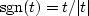Next: DAMPED OSCILLATION Up: CAUSAL INTEGRATION FILTER Previous: Symmetrical double integral

## Nonuniqueness of the integration operator

Integration can be thought of as.The implied division by zero atwarns us that this filter is not quite normal. For example,appears to be an imaginary, antisymmetric function of.This implies that the time function is the real antisymmetric signum function, namely,.The signum is not usually thought of as an integration operator, but by adding a constant we have a step function, and that is causal integration. By subtracting a constant we have anticausal integration. We can play games with the constant because it is at zero frequency that the definition contains zero division.

## EXERCISES:

1. Show that the mean of the input of leaky() is the mean of the output, which demonstrates that the gain of the filter is unity at zero frequency.Next: DAMPED OSCILLATION Up: CAUSAL INTEGRATION FILTER Previous: Symmetrical double integral
Stanford Exploration Project
10/21/1998